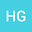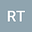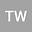Multiple nodal solutions for the Schr\”odinger-Poisson system with an asymptotically cubic term
•••Ronghua Tang
Hunan University of Science and Technology
Author ProfileTao Wang
Hunan University of Science and Technology
Author Profile## Abstract

This paper deals with the following Schr\“odinger-Poisson system \begin{equation}\label{zhaiyaofc}\left\{\begin{aligned} &-\Delta u+u+ \lambda\phi u=f(u)\quad\mbox{in }\mathbb{R}^3,\\ &-\Delta \phi=u^{2}\quad\mbox{in }\mathbb{R}^3, \end{aligned}\right.\end{equation} where $\lambda>0$ and $f(u)$ is a nonlinear term asymptotically cubic at the infinity. Taking advantage of the Miranda theorem and deformation lemma, we combine some new analytic techniques to prove that for each positive integer $k,$ system \eqref{zhaiyaofc} admits a radial nodal solution $U_k^{\lambda}$, which has exactly $k+1$ nodal domains and the corresponding energy is strictly increasing in $k$. Moreover, for any sequence $\{\lambda_n\}\to 0_+$ as $n\to\infty,$ up to a subsequence, $U_k^{\lambda_n}$ converges to some $U_k^0\in H_r^1(\mathbb{R}^3)$, which is a radial nodal solution with exactly $k+1$ nodal domains of \eqref{zhaiyaofc} for $\lambda=0$. These results give an affirmative answer to the open problem proposed in [Kim S, Seok J. Commun. Contemp. Math., 2012] for the Schr\”odinger-Poisson system with an asymptotically cubic term.
07 Jul 2021Submitted to Mathematical Methods in the Applied Sciences
07 Jul 2021Submission Checks Completed
07 Jul 2021Assigned to Editor
10 Jul 2021Reviewer(s) Assigned
19 Oct 2021Review(s) Completed, Editorial Evaluation Pending
20 Oct 2021Editorial Decision: Revise Minor